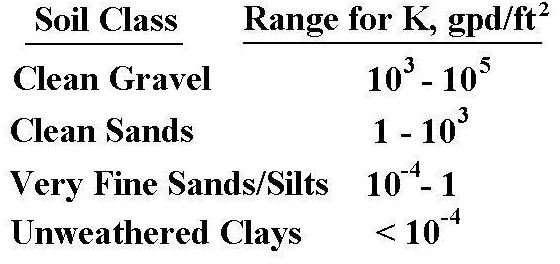# Hydraulic Gradient, Darcy's Law, and Groundwater Flow Modeling

Page content

## Introduction

Darcy’s Law is an empirical relationship for liquid flow through a porous medium. A common application is groundwater flow through an aquifer. Darcy’s Law gives the relationship among the flow rate of the groundwater, the cross-sectional area of the aquifer perpendicular to the flow, the hydraulic gradient, and the hydraulic conductivity of the aquifer.

## Darcy’s Law Equation

The equation for Darcy’s Law is based on the observations that the flow rate through a porous medium (such as an aquifer) is proportional to the cross-sectional area perpendicular to flow and is also proportional to the head loss per unit length in the direction of flow. Putting these two proportionalities together gives the following equation:

Q = KA(hL/L), where

Q = flow rate of liquid through the porous medium, typically in ft3/sec,

A = cross-sectional area perpendicular to flow, typically in ft2,

hL = head loss over a horizontal length, L, in the direction of flow (hL in ft and L in ft)

The diagram at the right shows an experimental apparatus illustrating the Darcy’s Law equation and its parameters.

Darcy’s Law is valid only for laminar flow, which occurs for Reynold’s number less than 1. Reynold’s number (Re) for flow through a porous medium is defined as: Re = ρVL/μ, where ρ and μ are the density and viscosity of the liquid, V is the flow velocity (Q/A), and L is a characteristic length, typically taken as the mean grain diameter of the medium. Most practical applications of groundwater flow have Re < 1, and thus can be modeled with Darcy’s Law.

The hydraulic grade line is shown in the diagram in the previous section. It is a line whose height above a flowing fluid is equal to the height water rises in a peizometer at that point, as shown in the diagram. That height of the water in the peizometer above the flowing liquid is equal to P/γ at any point in the flow field. The slope of the hydraulic grade line is hL/L and is often called the hydraulic gradient. The symbol i is often used to represent the hydraulic gradient. Then i = hL/L and Darcy’s Law can be given as Q = KAi.

## The Hydraulic Conductivity, KThe hydraulic conductivity, K, is a constant for a given porous medium. A very porous medium with little resistance to flow will have a high value for K, while a tightly packed medium with high resistance to flow will have a low value for K. The approximate range of K values for several types of soil are given in the table at the left.

From the units of the other parameters in the Darcy’s Law equation, one can see that K must have units of volumetric flow rate over area. In the table at the left the units are gpd/ft2. Other commonly used units for K are: ft/day (ft3/day/ft2) or m/day (m3/day/m2).

The values of K in the table are for the flow of water through the indicated porous media. The effect of the medium and the properties of the liquid flowing through it can be separated by the use of the specific pemeability, k, as shown in the following equation:

K = kγ/μ, where

k = specific permeability, ft2 (a property of the porous medium only),

γ = specific weight of flowing liquid, lb/ft3,

m = viscosity of flowing liquid, lb-sec/ft2,

K = hydraulic conductivity, ft/sec (ft3/sec/ft2).

Values of specific permeability are sometimes given with the darcy as the unit, where 1 darcy = 1.062 x 10-11 ft2.

## Analogy with Ohm’s Law and Fourier’s Law of Heat Conduction

It can be readily seen that Darcy’s Law is analagous to two other well-known empirical laws, Ohm’s law and Fourier’s Law of Heat Conduction, when all three are expressed in the following form: flow rate = driving force/resistance to flow. The three laws expressed in this form are given here:

Ohm’s Law: I = V/R (Current = voltage /resistance)

Fourier’s Law of Heat Conduction: Q = ΔT/(L/kA) {heat flow rate = temp. diff./[length in dir of heat flow/(thermal conductivity)(area normal to heat flow)]}

Darcy’s Law: Q = hL/(L/kA) {liquid flow rate = head loss/[length in dir of flow/(hydraulic conductivity)(area normal to flow)]}

The analogy between Darcy’s Law and Fourier’s Law of Heat Conduction is especially striking.